Russian Journal of Earth Sciences
Vol 1, No. 1, July 1998
Translated December 1998

# A Self-Consistent 2D Model of Mantle Convection with a Floating Continent

V. P. Trubitsyn and V. V. Rykov

Shcmidt United Institute of Physics of the Earth, Russian Academy of Sciences, B. Gruzinskaya ul. 10, Moscow, 123810 Russia

### Abstract

The mantle is modeled by a viscous fluid filling a horizontally elongated 2D region with an aspect ratio of 10:1. A model with Ra = 10 6 is constructed on a 200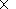80 mesh. Developed nonsteady-state thermal convection including narrow downwellings and upwellings sets in, with mantle flow velocities ranging from 1 to 10 cm/yr. Then, at a certain moment, a continent floating on the mantle is introduced into the model. The continent is modeled by a thin long plate of a thickness of 0.03 and a length of 2.0 relative units with respect to the mantle thickness, which corresponds to dimensional values of 90 and 6000 km, respectively. To demonstrate mantle heating beneath continent, the latter is positioned at the coldest place of the mantle where downgoing flows dominate at the moment chosen. The evolution of the mantle-continent system is found from numerical solution of equations governing the momentum, mass, and heat transfer in viscous fluid and rigid continent. The problem is rigorously formulated, a self-consistent method is given for the solution of coupled integrodifferential equations, and a technique of their numerical implementation is described. The continent remains virtually immobile during a long time (about 500 Ma), but the mantle flow pattern dramatically changes, which results in suppression of cold mantle downwellings under the continent and their gradual replacement by hot upwellings. Afterwards the viscous drag of mantle flows begins to move the continent at a variable velocity averaging about 1 cm/yr. The mantle flow pattern and continent velocity constantly changes under the action of mechanical coupling and thermal interaction between the mantle and moving continent. After a time of about 1.5109, when the continent has traveled over a distance of about 15000 km, it arrives at a place where several cold mantle downwellings concentrate. Then the continent velocity sharply decreases, and the continent continues its motion in either primary or opposite direction, depending on the general mantle flow pattern. The results of the numerical experiment can be used for the analysis of mechanism responsible for the motion of Eurasia-type continents, origination and ascent of plumes, and geodynamic processes in the subcontinental mantle.

### 1. Introduction

Presently, various numerical experiments have been used for the study of thermal convection in the Earthşs mantle. The role of variable, temperature-dependent viscosity, pressure and temperature, as well as the effect of phase transformations on the mantle flow pattern, have been examined in terms of 2D and 3D models in Cartesian and spherical coordinates (see e.g. [Christensen, 1983, 1984; Christensen and Yuen, 1984; Glatzmaier, 1988; Machetel and Weber, 1991; Lenardic and Kaula, 1994; Solheim and Peltier, 1994; Tackley et al., 1994; Parmentier et al., 1994]). Numerical modeling has provided an insight into many basic regularities of global geodynamics. In the nearest decade, a numerical modeling problem of vital importance is the construction of self-consistent models in which oceanic high-viscosity lithosphere breaks into separate plates.

Another important problem is the exploration of the effect of continents on the mantle convection structure. Continents cover more than a quarter of the Earth's surface. The mantle heat flow in continental areas is about 30 mW/m 2, which is one-third as much as the heat flow in oceanic areas, averaging 90 mW/m 2. This is explained by the fact that continents are thermal insulators of the mantle, because only the conductive mechanism of heat transfer is operative within continents. Various estimates of the Nusselt number, characterizing the whole-mantle convective heat loss, give a value of about 20. The oceanic lithosphere, involved in the convective circulation of matter, virtually remains within the convective thermal boundary layer and, despite its high viscosity, cannot serve as an effective thermal screen for the mantle. Thus, even immobile continents should considerably affect the structure of mantle convection.

Based on a simplified model, Christensen,  compared the mantle flow patterns beneath continents and oceans. Trubitsyn and Fradkov,  showed that thermal convection in the upper mantle is suppressed, resulting in a threefold decrease in the continental heat flow. As was shown in later works [Trubitsyn et al., 1993a, 1993b, 1994; Trubitsyn and Bobrov, 1993; 1996, 1997; Lowman and Jarvis, 1993, 1995, 1996; Bobrov and Trubitsyn, 1996; Nakakuki et al., 1997], an immobile continent initially suppresses the underlying mantle convection and broadens the convective cell; afterwards, when the subcontinental mantle had been heated during the following few hundreds of millions of years, a hot upwelling mantle flow develops beneath the continent.

Since continents are not fixed but float on the mantle, their effect on the mantle convection structure is even greater. In early studies, the effect of continental drift was included in a simplified form, as an effective boundary condition. In continental areas, the free upper boundary condition was replaced by fixed values of horizontal velocity [Lux et al., 1979]. In a similar manner, Doin M.-P.,  simulated the effect of moving continents by a time-dependent velocity specified at the upper boundary.

Gurnis,  presented the results of a consistent numerical 2D model of mantle convection with free floating continents. For the first time, a numerical 3D model of mantle convection with two floating continents was constructed by Trubitsyn and Rykov,  and Rykov and Trubitsyn, 1996a, 1996b]. This model reconstructs general regularities of the formation and breakup of Pangea [Trubitsyn and Rykov, 1995]. Structures similar to the Atlantic and Pacific oceans form upon the Pangea breakup. In terms of this model, a very steep subduction zone (of the Kuril-Kamchatka type) develops at one of the Pacific margin, and a very gently dipping subduction zone (of the South American type) develops at the other. Assuming an alternative initial position of continents, Rykov and Trubitsyn, [1996b] obtained a structure consisting of two coupled continents (similar to North and South Americas), which developed upon the breakup of a supercontinent.

Trubitsyn and Rykov,  developed a self-consistent numerical model of low-Ra convection in the upper mantle having a variable viscosity and interacting with a moving continent modeled by a thick rigid plate. The cases with a free plate floating over the mantle and moving at a prescribed fixed velocity were considered. The continent approaching a downgoing cold mantle flow was found to deflect this flow and form structures similar to inclined subduction zones. The dip angle of the downgoing mantle flow decreases with the velocity of the approaching continent.

This work describes in detail the mathematical formulation of the problem and the method of its solution. A Ra = 10 6 convection model close to the real Earth is constructed on a 20080 mesh, including a thin continent of thickness d = 90 km and horizontal size l = 6000 km. The long-term evolution of the mantle-continent system is calculated. Comparison of the evolution of unsteady-state convection in the mantle with and without a continent shows that the moving continent dramatically changes the mantle convection structure.

### 2. Model

The mantle is modeled by an incompressible constant-viscosity fluid occupying the volume of an elongated rectangular 2D layer of a thickness D and length L, with the aspect ratio L:D=10:1. The upper boundary is assumed to be free and other boundaries are impermeable, with a slip condition. The lower and upper boundaries have fixed temperatures T=T0 and T=0, respectively; i.e. the layer is heated from below. The side walls are thermally insulated. The coordinate origin coincides with the left-hand lower corner, the z axis is directed upward, and the x axis is directed to the right.

Continents are represented as light rigid rectangular thick plates that are heat conductive, float in the mantle, and have the length l and thickness d+d0, where d is the depth to which the continent is immersed in the mantle and d0 is the continent elevation above the mantle. The viscous coupling with mantle flows drives the continent toward downgoing mantle flows. Because the heat transfer mechanism within continents is purely conductive, the continents produce a heat insulating effect which prevents heat to escape from the mantle. Also, the continents affect mechanically the mantle, because the no-slip condition tends to attenuate velocity contrasts in mantle flows adjacent to the lateral and lower boundaries of the continents.

### 3. The System of Equations of Mantle Convection with Floating Continents

#### 3.1. Equations of thermal convection

Thermal convection in a viscous mantle is described by the distributions of convective vector velocities Vi(x, y, z), temperature T(x, y, z), and pressure p(x, y, z). These unknown functions are found from the solution of three equations of momentum, heat, and mass transfer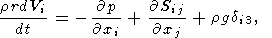(1)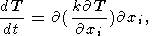(2)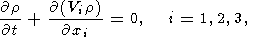(3)

where r is density, g is gravity, T is temperature measured from the adiabatic distribution, k is diffusivity, dij is the Kronecker delta, and Sij is the deviator tensor of viscous stresses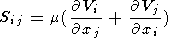(4)

Here m is viscosity. The relative values of inertial terms in the left-hand side of mantle momentum transfer equation (1) are of the order of kr /m10-23 with respect to the right-hand terms. Therefore, these inertial terms may be neglected.

We put r = r0(1 - a) in the buoyancy term of equation (1) and r = r0 in other terms of equations (1)-(2). Hereinafter the pressure is measured from its hydrostatic distribution p0(z) determined from the condition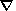p = -r0g. Also, we introduce dimensionless variables taking, as measurements units, the mantle thickness D for length, k/D for velocity, D2/k for time, T0 for temperature, m for viscosity, and mk/D2 for pressure and stresses.

Using these variables, 2D model equations of convection (2)-(4) take the form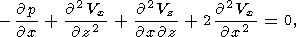(5)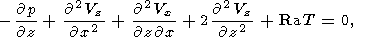(6)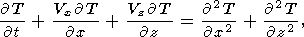(7)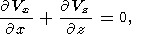(8)

where Ra is Rayleigh number,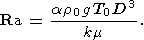(9)

#### 3.2. Equations of motion of a continent

In a 2D model, velocities u=(ux, uy) at all points of a rigid continent floating in the mantle (along its horizontal surface) have the same values and are equal to the velocity of its center of gravity: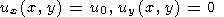(10)

The continents move under the action of viscous forces of mantle flows. The Euler equations of horizontal movement of a rigid continent have the form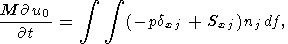(11)

where df is the elementary area of the surface, nj is the unit vector of outward normal to the area, and M is the dimensionless mass of the continent, referred to the unit length of the y axis in the 2D model considered. The integral is taken over the whole surface of the continent portion immersed in the mantle, which includes its base (z=1-d) and side walls ( x=x1 and x=x1+l ):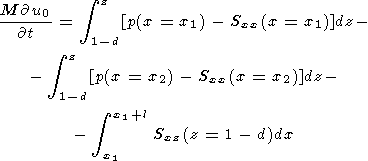(12)

where d and l are thickness and length of the immersed portion of continent; x1(t) and x2(t)=x1(t)+l are instantaneous coordinates of the left- and right-hand walls of the continent, which obey the conditions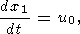(13)

The equation governing the temperature distribution Tc within the rigid continent in the initial fixed system of coordinates is reduced to the equation of heat conductivity with advective heat transfer controlled by the continent velocity u0 along x axis,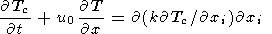(14)

Similar to the mantle, the relative value of inertial terms in the left-hand side of the equation of continent motion (12) is of the order of kr/m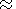10-23 compared to the terms in its right-hand side. Substituting deviator tensor of viscous stresses (4) into (12) and omitting inertial terms, we obtain the equations of continent motion in dimensionless variables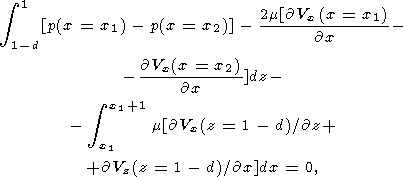(15)

where the coordinate x1 in the integration limits is determined from (13).

#### 3.3. Boundary conditions

Equations of mantle convection (6)-(10), continent motion (15) and (13), and heat transfer within the continent (14) are interconnected through boundary conditions.

Temperature boundary conditions at the lower and lateral boundaries of the whole area studied have the form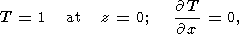(16)

at x=0 and x=10.

The mantle temperature at the free upper, z=1, surface vanishes (( T=0 ) only in the oceanic area, outside the continent, namely ( x < x1(t) and x > x1(t)+l ).

At the continental surface immersed in the mantle, the temperature and heat flow are set to be continuous between the mantle and continent; i.e.,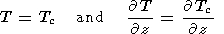(17)

at the base of the continent, z=1-d and x1(t) < x < x1(t)+l, and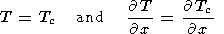(18)

at its lateral surface sections, 1-d < z < 1 and x = x1 or x = x2.

Zero temperature is set on the upper surface of the continent, i.e., Tc = 0 at x1(t) < x < x1(t)+l and z=1 + d0, where d0 is the elevation of the continent above mantle surface (commonly, d0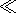d ).

As noted above, impermeability and slip conditions are imposed on mantle flows on the lower and lateral boundaries of the entire calculated region. Therefore, the lower boundary condition is Vz = 0, Sxz = 0 at z = 0. Because it is valid at all x, we have Vz/x = 0 at z = 0. In view of (4), we obtain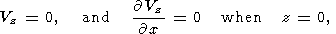(19)

Similar conditions are imposed on the lateral boundaries,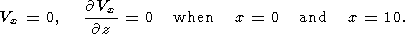(20)

Condition (19) is also imposed on the free upper surface z =1 outside the continent: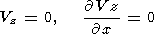(21)

at x < x1(t) and x > x1(t)+l.

The non-slip condition on the interface between the viscous mantle and moving continent implies that the velocities of mantle flow and continent motion coincide on this boundary: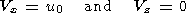(22)

at the base of the continent for x1(t) < x < x1(t) + l and z = 1 - d and at its wall sections for 1-d < z < 1, x = x1 and x = x2.

Conditions (22) simplify the equation of continent motion. Since the impermeability condition is satisfied all along the base of the continent irrespective of x, we also have Vz = 0 at the base. Consequently, the last term under the integral sign in (15) vanishes. Likewise, since the no-slip condition Vz = 0 is satisfied all over the walls of the continent irrespective of z, we have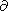Vz/z=0. Then, incompressibility condition (8) implies thatVx/x=0 on the walls. As a result, the equations of continent motion assume a simple form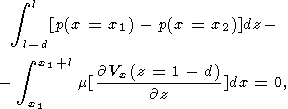(23)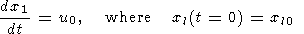(24)

Finally, the resulting mathematical problem may be stated as follows. In all, there are seven unknown functions. These are four position- and time-dependent functions for the mantle convection: two velocity components of mantle flows Vx(x, z, t) and Vz(x, z, t), temperature distribution T(x, z, t), and pressure distribution p(x, z, t), and three functions for the continent: temperature distribution within the continent Tá(x, z, t), instantaneous translational velocity of the continent as a whole u0(t), and the coordinate of its left-hand edge x1(t). These functions can be found from a closed system of seven coupled equations: four differential equations of convection (5)-(8), thermal conductivity equation for the continent (14), and the equation of motion of the rigid continent, which is reduced to the condition imposed on mantle flow velocity derivatives (23) and to relation (24) between the velocity of the continent and its position. The constants of integration of the differential equations are found from boundary conditions (18)-(22).

The difference between our problem with a free floating continent and the well-known problem with a fixed continent consists in the fact that the impermeability and no-slip conditions on the upper surface must be satisfied at the place currently occupied by the floating continent whose velocity and position are not a priori known but should be defined by solving the system of coupled differential equations at each time step.

### 4. Numerical Method

Two basically different methods are applicable to the solution of the system of thermal convection equations including floating continents. In the two-region method [Trubitsyn and Bobrov, 1996, 1997], equations of heat and mass transfer are solved at each time step separately outside and within the continent, and continuity conditions are then set at the interface between continent and mantle. In the one-region region [Trubitsyn and Rykov, 1995; Rykov and Trubitsyn, 1996a, 1996b], computations are conducted in a single coherent domain and explicitly incorporate the jump in material properties between mantle and continents on the surface of the continent.

The numerical algorithm solving the system of thermal convection equations including floating continents can be summarized as follows. Let convective flow velocities and mantle distributions of temperature T(t1) and pressure p(t1), as well as position x1(t1) and velocity u0(t1) of the continent, be known at a time moment t1. We have to find the solution of system (5)-(8), (14), (23) and (24) at the next time moment t2 = t1 + Dt. The new position of the continent x1(t2) at the moment t2 is readily found from (24): x1(t2) = x1(t1) + u0(t)1)Dt. If the continent velocity at this moment u0(t2) were also known, one could solve thermal convection equations (5)-(8) with boundary conditions for temperature (18) and velocities (19)-(22), consistent with the new position of the continent, and find mantle flow velocities Vx(t2) ¨ Vz(t2), temperature T(t2) and pressure p(t2) at the time moment t2. However, the complexity of the problem lies just in the fact that the velocity of the continent u0(t2) is unknown. Its value must comply with mantle flow velocities Vx(t2) and Vz(t2) that obey equation of continent motion (24). Therefore, an iterative technique should be developed for determining this velocity of the continent. In principle, based on continent velocity values chosen in accordance with a specified enumeration procedure, convective velocity fields and integrals in (23) can be calculated until a value of u0(t2) is found for which the right-hand side of (23) deviates from zero by a quantity e complying with desired accuracy. Since the right-hand side physically represents the force that acts on the continent, we have e > 0 if the chosen velocity of the continent u0(t2) is underestimated and e< 0 if it is overestimated.

A finite difference method was used for solving the system of equations of thermal convection including floating continents [Rykov and Trubitsyn, 1996b]. Numerical solution of temperature transfer equation (7) or (14) employed the flux-corrected transport method of Zalesak, . Equations for velocities and pressure (5), (6) and (8) were reduced to elliptic equations with variable coefficients (generalized Poisson equations). They were solved with the help of the three-layer triangular method with a conjugate-gradient choice of iteration parameters [Samarskii and Nikolaev, 1978].

### 5. Numerical Results

#### 5.1. Free nonsteady-state mantle convection

The mantle was modeled by a viscous fluid that occupies an elongated 2D region. Given the mantle thickness D3000 km, its outer circumference 2pR40000 km, and its inner circumference 2p (R-D)21000 km, the region approximating the mantle had an aspect ratio of L:D = 10:1. The simplest, Ra= 106 model with heating from below was computed on a 20080.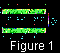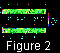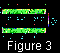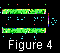Figures 1a, 2a, 3a, and 4a present model patterns of the mantle thermal convection developed after the dimensionless time moment t =1.0995. As is known, the whole region, even with no convection, is heated throughout at t > 1. Therefore, we may consider the convection as well-developed by that moment. However, at Ra > 2105 thermal convection is nonstationary and quasi-turbulent, because the nonlinear terms VxT/x and VzT/z in (7) generate new, smaller-degree harmonics at each time step. With increasing Rayleigh number, these nonlinear terms become larger, and smaller-scale time-variable inhomogeneities arise as a result of self-organization.

The dimensionless temperature equal to T=0 at the upper surface and to T0=1 at the lower one is shown in the figure by variable shades. The magnitude and direction of the mantle flow velocity at each point are indicated by an arrow. Velocity scales are shown in the lower right-hand corners of the figures. Maximum dimensionless values of the velocities are V max1500.

The thin (red in the on-line color figure version) line in the upper part of the figures shows the inferred distribution of the Nusselt number (dimensionless heat flow q = -T/z ) over the external surface of the region. The mean Nusselt number is Nu17. The amplitude of relative variations in the heat flow is about 100%.

The thick (green in the on-line color figure version) line shows the inferred topography, i.e., deformations of the upper free surface arising under the action of viscous convection stresses. The scale of dimensionless relief elevations multiplied by a factor of 10-3 is shown on the right-hand axis. The elevations were estimated from the formula h = Szz/rg. Taking into account the stress unit defined in Section 3, the relief elevation unit is mk/(rgD2). As seen from Figures 1a, 2a, 3a, and 4a, mean amplitudes of the dimensionless elevations are of an order of 25103.

Setting, for the Earth, D3 106 m, a3 10-5 K-1, DT2 103 K, k10-6 m2 s-1, m1022 Pa s, r5 103 kg/m3, and g10 m s-2, the time and velocity units can be found. The velocity unit is k/D10-5 m/yr, and the time unit is t=D2/k31011 years. According to (9), the Rayleigh number, which characterizes the intensity of mantle thermal convection, is Ra8 106.

Since the above parameters of the Earth are not accurate enough, we used an approximate Rayleigh number Ra = 106. Since mantle flow velocities obey the proportionality relation VRa 2/3 [Turcotte and Schubert, 1982], the velocities and times can easily obtained for somewhat different Rayleigh numbers. With the Earth parameters specified above, the inferred results and observed data may be compared if the velocity values are multiplied by 82/3 = 4 and the time is divided by 4. As a result, maximum mantle flows have V max1500410-5 m/yr6 cm/yr.

Figures 1a, 2a, 3a, and 4a illustrate the inferred evolution of mantle convection over a large time interval. The dimensionless times are indicated in the left-hand parts of the figures. As noted above, the unit time is t31011 years. In order to calculate the time interval between the convection states shown in Figures, the dimensionless times indicated in the figures should be multiplied by 31011 years and divide by 4 (due to Rayleigh number rescaling); i.e., the dimensionless times should be multiplied by 75109 years. Then, the overall evolution time of mantle convection shown in Figures is (1.1495 - 1.0995)75109 years3.6109 years.

The relief elevation unit is mk/(rgD2)0.02 m so that dimensionless amplitudes of inferred relief elevations of 2.5104 correspond to 0.5 km.

5.2. Evolution of the mantle convection with a floating continent

Figures 1b, 2b, 3b, and 4b present the inferred mantle convection patterns including thermal and mechanical interaction effects with a moving continent. For comparison, Figures 1a, 2a, 3a, 4a and Figures 1b, 2b, 3b, 4b show the patterns calculated at the same time moments. A continent represented by a rigid plate of the thickness d=0.03 D=90 km and length l=2 D= 6000 km is introduced at the time moment t=1.0995. In Figures 1b, 2b, 3b, and 4b, the continent is shown by gray. It is intentionally chosen to initially occupy the coldest place in the mantle where outgoing mantle flows are most abundant. The figures show that, due to the heat-insulating effect, the mantle under the continent is gradually heated. By the time t=1.11511.2109 years, a well-defined system of hot mantle upwellings has formed under the continent, and the continent starts drifting to the right under the action of mantle flow viscous drag force. Since the continent at each moment interacts with mantle flows, the mantle convection structure changes as the continent moves. The dimensionless continental drift velocity first increases from 100 to 400 and then decreases. According to the definitions made above, dimensional velocities range from 0.4 to 1.6 cm/yr. As seen from Figures 1b, 2b, 3b, and 4b, after two billion years, when the continent had traveled a distance of about 5 D15000 km, it nearly stops.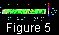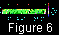In the on-line version of this paper, Figures 1a, 2a, 3a, 4a and 1b, 2b, 3b, 4b are complimented by movies (Figure 5 and Figure 6, respectively), each including 30 frames.

### 6. Conclusion

The evolution of free unstable-state mantle convection and evolution of mantle convection coupled with a free floating continent were calculated. The comparison of concurrent distributions of temperature and mantle flow velocities derived from these two models has demonstrated that floating continents dramatically change the structure and evolution of mantle convection. Detailed evolution results presented in this paper are useful for investigations into the driving mechanism of Eurasia-type continent motion, plume origination and upwelling, and thermal regime of subcontinental mantle.

#### Acknowledgments

This work was supported by the International Center of Research and Technology, Grant No. 415-96 and the Russian Foundation for Basic Research, Project No. 96-05-66069.

### References

Bobrov A. M., Trubitsyn V. P., Physics of the Earth, N. 7, 1995, p. 5-13 (in Russian).

Bobrov. A. M., Trubitsyn V. P., Times of rebuilding of mantle flows beneath continent, Izvestiya, Physics of the Solid Earth, 31, 1996, pp. 551-559.

Christensen U., A numerical model of coupled subcontinental and oceanic convection, Tectonophysics, 95, 1983, pp. 1 - 23.

Christensen U. R., Convection wih pressure- and temperature-dependent non-Newtonian rheology, Geophys. J. Astr. Soc., 77, 1984, pp. 343-384.

Christensen U. R. and Yuen D. A., The interaction of a subducting lithospheric slab with a chemical or phase boundary, J. Geophys. Res., 89, 1984, pp. 4389-4402.

Doin M.-P., Fleitout L. and Christensen U., Mantle convection and stability of depleted and undepleted continental lithosphere, J. Geophys. Res., 102, 1997, pp. 2771-2787.

Glatzmaier G. A., Numerical simulation of mantle convection: Time-dependent, three-dimensional, compressible, spherical shell, Geophys. astrophys. Fluid Dyn., 43, 1988, pp. 223-264.

Guillou, L. and Jaupart C., On the effect of continents on mantle convection, J. Geophys. Res., 100, 1995, pp. 24217-24238.

Gurnis M., Large-scale mantle convection and aggregation and dispersal of supercontinents, Nature, 332, 1988, pp. 696-699.

Gurnis M. and Zhong S., Generation of long wavelengh heterogeneitiey in the mantle dynamics interaction between plates and convection, Geophys. Res. Lett., 18, 1991, pp. 581-584.

Lenardic A. and Kaula W. M., Tectonic plates, D " thermal structure, and the nature of mantle plumes, J. Geophys. Res., 99, 1994, pp. 15697-15708.

Lowman J. P. and Jarvis J. T., Mantle convection flow reversals due to continental collisions, Geophys. Res. Lett., 20, 1993, pp. 2091-2094.

Lowman J. P. and Jarvis J. T., Mantle convection models of continental collision and breakup incorporating finite thickness plates, Phys. Earth Planet. Inter., 88, 1995, pp. 53068.

Lowman J. P. and Jarvis J. T., Continental collisions in wide aspect ratio and high Rayleigh number two-dimensional mantle convection models, J. Geophys. Res., 101, B11, 1996, pp. 25485-25497.

Lux R. A. Davies G. F. and Thomas J. H., Moving lithospheric plates and mantle convection, Geophys. J. R. Astron. Soc., 57, 1979, pp. 209-228.

Machetel P. and Weber P., Intermittent layered convection in amodel mantle with an endothermic phase change at 670 km, Nature, 350, 1991, pp. 55-57.

Nakakuki T. Yuen D. A. and Honda S., The interaction of plumes with the transition zone under continents and oceans, Earth Planet. Sci. Lett., 146, 1997, pp. 379-391.

Parmentier E. M. Sotin C. and Travis B. J., Turbulent 3-D thermal convection in an infinite Prandl number, volumetrically heated fluid; Implication for mantle dynamics, Geophys. J. Int., 116, 1994, pp. 241-254.

Rykov V. V., Trubitsyn V. P., Computational seismology, v. 26, Geodynamics and Earthquake Prediction, 1994, p. 94-102 (in Russian).

Rykov V. V., Trubitsyn V. P., Computational seismology, v. 27, Theoretical Problems of Geodynamics and Seismology, 1994, p. 21-41 (in Russian).

Rykov V. V. and Trubitsyn V. P., Numerical technique for calculation of three-dimensional mantle convection and tectonics of continental plates, in Computational Seismology and Geodynamics, v. 3 ed. by D. K. Chowdhury. Am. Geophys. Un., Washington D.C. 1996a. pp. 17-22. (English translation of Vychisliteljnaya seismologiya, Nauka, M., No. 26, 1994, pp. 94-102)

Rykov V. V and Trubitsyn V. P., 3-D model of mantle convection incorporating moving continents, in Computational Seismology and Geodynamics, v. 3 ed. by D. K. Chowdhury. Am. Geophys. Un., Washington D.C., 1996b, pp. 23-32. (English translation of Vychisliteljnaya seismologiya, Nauka, M., No. 27, 1994, pp. 21-41)

Samarsky A. A., Nikolayev E. C., Methods of Solving Residual Equations, Nauka, M., 1978, 591 pp. (in Russian).

Samarskii A. A. and Nikolaev E. S., Method of solving finite-difference equations (in Russian), Nauka, M., 1978, 591 p.

Solheim L. P. and Peltier W. R., Phase boundary deflections at 660-km depth and episodically layered isochemical convection in the mantle, J. Geophys. Res., 99, 1994, pp. 15861-15875.

Tackley P. J., Stevenson D. J., Glatzmaier G. A., Schubert G., Effect of multiple phase transitions in three dimension spherical model of convection in Earth's mantle, J. Geophys. Res., 99, 1994, pp. 15877-15901.

Trubitsyn V. P., Belavina Yu. F., Rykov V. V., Physics of the Earth, N. 11, 1993, p. 3-13 (in Russian).

Trubitsyn V. V., Belavina Yu. F., Rykov V. V., Thermal and mechanical ineraction of mantle and continental lithosphere, Izvestiya, Physics of the solid Earth, 29, 1993, pp. 933-945. (English translation of Fizika Zemli, No. 11, 1993, pp. 3-13. Published by American Geophysical Union and Geological Society of America).

Trubitsyn V. P., Belavina Yu. F., Rykov V. V., Physics of the Earth, N. 7/8, 1994, p. 5-17 (in Russian).

Trubitsyn V. V., Belavina Yu. F., Rykov V. V., Thermal mantle convection in a varying viscosity mantle with a finite-sized continental plate, Izvestiya, Physics of the solid Earth, 30, 1995, pp. 587-599. (English translation of Fizika Zemli, No. 7/8, 1994, pp. 5-17. Published by American Geophysical Union and Geological Society of America).

Trubitsyn V. P., Bobrov A. M., Physics of the Earth, N. 9, 1993, p. 27-37 (in Russian).

Trubitsyn V. P., Bobrov A. M., Kubyshkin V. V. Physics of the Earth, N. 5, 1993, p. 3-11 (in Russian).

Trubitsyn V. P., Bobrov A. M., and Kubyshkin V. V., Influence of continental lithosphere on structure of mantle thermal convection, Izvestiya, Physics of the solid Earth, 29, 1993, pp. 377-385. (English translation of Fizika Zemli, No. 9, 1993, pp. 27-37. Published by American Geophysical Union and Geological Society of America).

Trubitsyn V. P., Bobrov A. M., Computational seismology, v. 27, Theoretical Problems of Geodynamics and Seismology, 1994, p. 3-20 (in Russian).

Trubitsyn V. P., Bobrov A. M., Computational seismology, v. 28, Modern Problems of Seismicity and Earth Dynamics, 1996, p. 22-31 (in Russian).

Trubitsyn V. P. and Bobrov A. M., Structure evolution of mantle convection after breakup of supercontinent, Izvestiya, Physics of the solid Earth, 29, 1994, pp. 768-778. (English translation of Fizika Zemli, No. 9, 1993, pp. 27-37. Published by American Geophysical Union and Geological Society of America).

Trubitsyn V. P. and Bobrov A. M., Thermal and mechanical interaction of continents with the mantle, in Computational Seismology and Geodynamics, v. 3, ed. by D. K. Chowdhury. Am. Geophys. Un., Washington D.C, 1996, pp. 33-41. (English translation of Vychisliteljnaya seismologiya, Nauka, M., No. 27, 1994, pp. 3-20).

Trubitsyn V. P and A. M. Bobrov A. M., Structure of mantle convection beneath stationary continents, in Computational Seismology and Geodynamics, v. 4, ed. by D. K. Chowdhury. Am. Geophys. Un., Washington D.C., 1997, pp. 42-53. (English translation of Vychisliteljnaya seismologiya, Nauka, M., No. 28, 1996, pp. 22-31).

Trubitsyn V. P., Fradkov A. C., Physics of the Earth, N. 7, 1985, p. 3-14 (in Russian).

Trubitsyn V. P. and Fradkov A. S., Convection under continnts and oceans, Izvestiya, Physics of the solid Earth, 21, No. 7, 1985, pp. 491-498. (English translation of Fizika Zemli, No. 7, 1985, pp. 3-13. Published by American Geophysical Union and Geological Society of America).

Trubitsyn V. P. and Rykov V. V., A 3-D numerical model of the Wilson cycle, J. Geodynamics, 20, 1995, pp. 63-75.

Trubitsyn V. P., Rykov V. V., Physics of the Earth, N. 6, 1997, p. 1-12 (in Russian).

Trubitsyn V. P. and Rykov V. V., Mechanism of formation of an inclined subduction zone, Izvestiya, Physics of the Solid Earth, 33, No. 6, 1997, pp. 427-437. (English translation of Fizika Zemli, No. 6, Interperiodica Publishing, 1997, pp. 1-12).

Turcotte D. L. and Schubert G., Geodynamics: Applications of Continuum Physics to Geological Problems, John Wiley, New York, 1982, 449 pp.

Zalesak S. T., Fully multidimensional flux-correced transport algorithms for fluids, J. Comp. Phys., 31, 1979, pp. 335-361.

Zhong S. and Gurnis M., Dynamic feedback between a continentlike raft and thermal convection, J. Geophys Res., 98, 1993, pp. 12219-12232.

Zhong Sh. and Gurnis M., Role of plates and temperature-dependent viscosity in phase change dynamics, J. Geophys. Res., 99, 1994, pp. 15,903-15,917.

Load files for print and local use.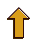This document was generated by TeXWeb (Win32, v.1.0) on February 23, 1999.